# Analyzing Goos–Hänchen Shift on Dielectric Gratings

## Introduction

When a beam experiences a total internal reflection, a lateral shift exists between the reflected

and the incident beams.  It is called Goos and Hänchen effect, named after its discoverers.  For decades, the phenomenon has been a research topic attracting considerable amount of efforts for its intriguing physical nature. It gained renewed attention in recent years when plasmonic materials

and metamaterials are involved.  Sensor schemes based on GH shift has also been proposed, and have led to sensors of biochemical, thermal and wavelength monitoring applications.  The sensitivity in these sensors are directly related to the amount of GH shift, thus, to achieve a large GH shift (positive or negative) is of practical interests.

In reference paper , the possibility to use the guided mode resonance to achieve giant GH shift was proposed.  As shown in Figure 1, the incidence comes from the substrate side of the grating at an angle greater than the critical angle of the substrate and will be totally reflected from the surface of the substrate decorated by the dielectric grating. When the incidence couples to the leaky mode of the grating, strong energy flow inside the waveguide exists, thus giant GH shift could be realized. When the leaky mode is a negative propagating one (i.e. the energy flow is anti-parallel to the wave vector of the mode), negative GH shift is expected.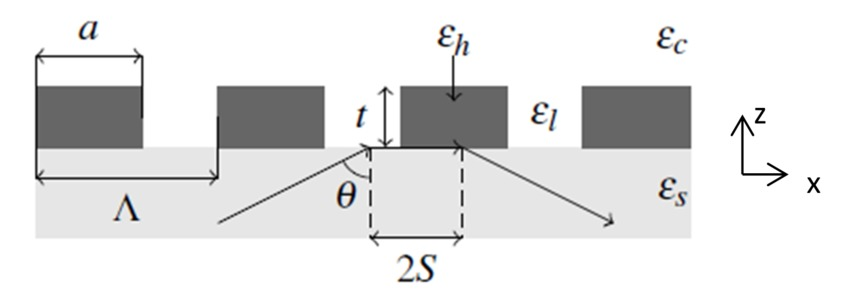Figure 1. Schematic of a dielectric grating and the GH shift under a total internal reflection. The grating specs are  Γ = a/ Λ (duty cycle),  Λ = 0.43um, Γ = 0.93, t = 0.11um, ɛh = 12.12,  ɛl = ɛc = 1(free space), ɛs = 2.09(SiO2).

The GH shift is defined as: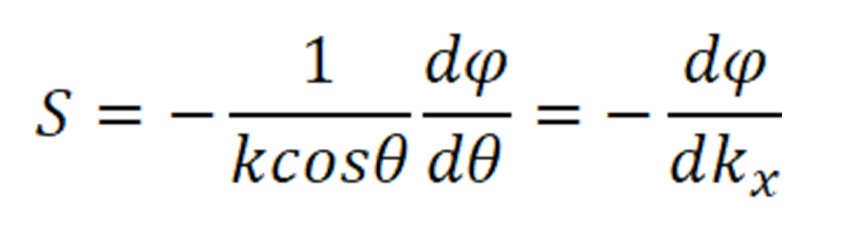Here Ɵ is the incident angle of a plane wave from substrate side; ɸ is the phase of the reflection coefficient; k is the wavenumber inside the medium of the incident side, while kx =k sin(Ɵ).

To study the GH shift, the incidence is of s polarization with the electric field perpendicular to the plane of incidence.  k0 and kx are selected that inside the substrate only the 0th order diffraction (the direct reflection) is propagating, while all higher order diffraction modes are evanescent, while in the air both the 0th and higher diffraction orders are evanescent.  For 1.5um free space wavelength of the incidence, this corresponds to kx between 0.287 ∼ 0.414×2 π/ Λ, or Ɵ between 43.8∼ 90.  Our RCWA program DiffractMOD can be used here to measure the 0th diffraction order phase vs kx  by varying incident angle.  The design files can be accessed on the Customer Support Portal (account required).

The structure with above grating specs is set up in Synopsys Rsoft CAD, as shown in Figure2.  Only one period is needed to be drawn with RCWA simulation.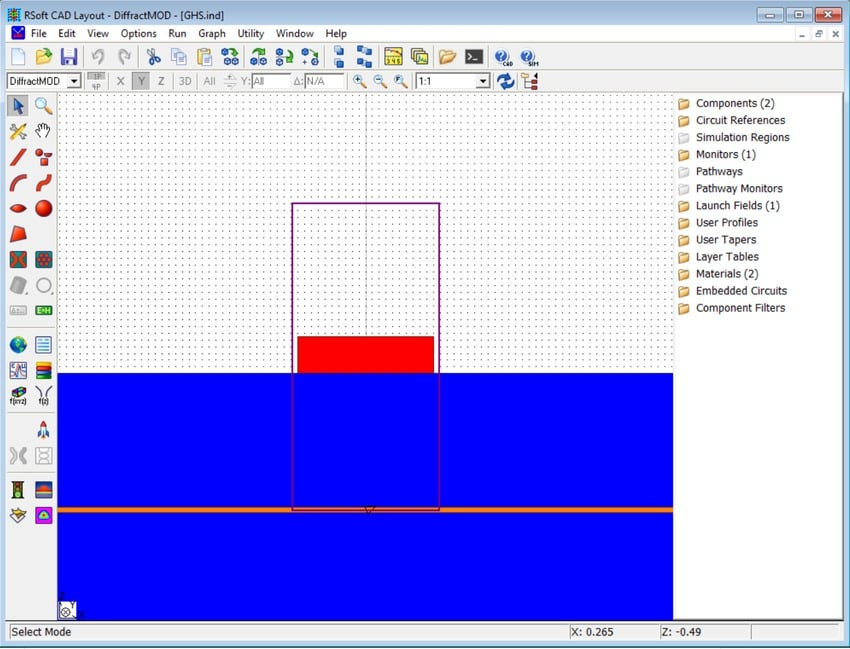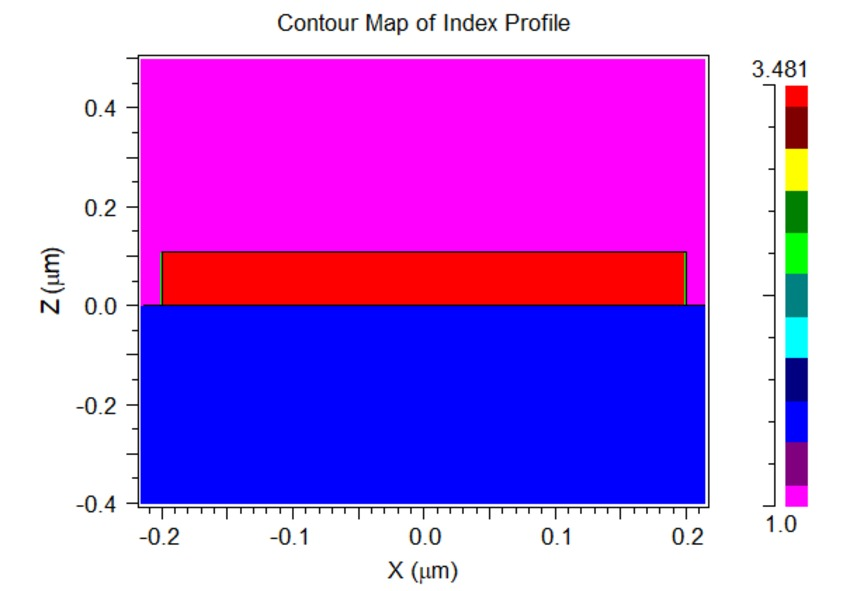Figure 2. Structure set up in RSoft CAD Environment (left) and index distribution (right) for one grating period.

## Simulation Procedure

1. Complex E Field coefficient of reflection for s incident is measured.
2. Scan kx  via changing incident angle with MOST.
3. Define a user measurement to extract the phase of the 0th order from complex field coefficient data file.
4. Unwrap phase data file with python unwrap function.
5. Use “mathmat” utility to form a data file with unitless phase vs kx.
6. Use “disperse” utility to calculate the first derivative of -dɸ/dkx .
The simulation results are shown Figure 3. The curve of the reflection coefficient phase exhibits a sharp increase of almost 2π at around kx = 0.38×2π /L, or q = 67.0. A sharp dip in the curve of GH shift is observed at the same kx value when the phase exhibits a steep increase. At its maximum magnitude, the GH shift reaches a value of over -1000um. Figure4 shows the electric field and Ponyting vector distribution at kx = 0.38×2π /L. At this kx point, the field goes to +x direction but the energy points to the opposite direction.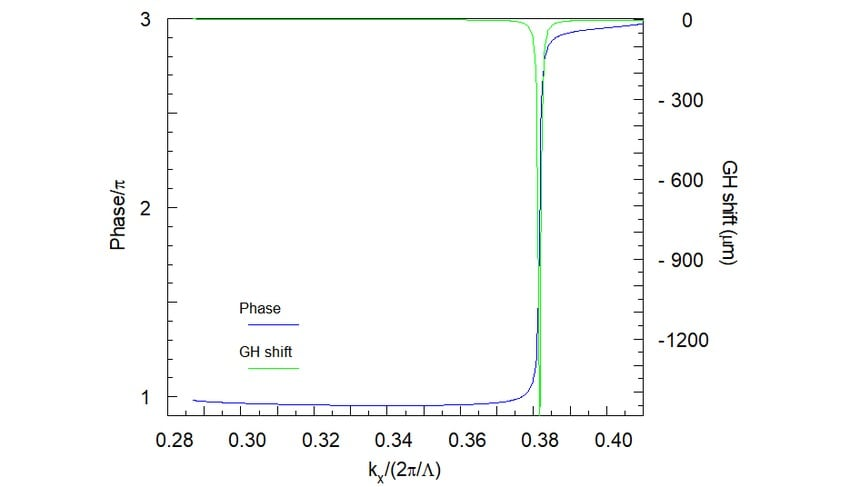Figure 3. Phase of the reflection coefficient and GH shift vs kx for an incidence of 1.5um free space wavelength from the substrate.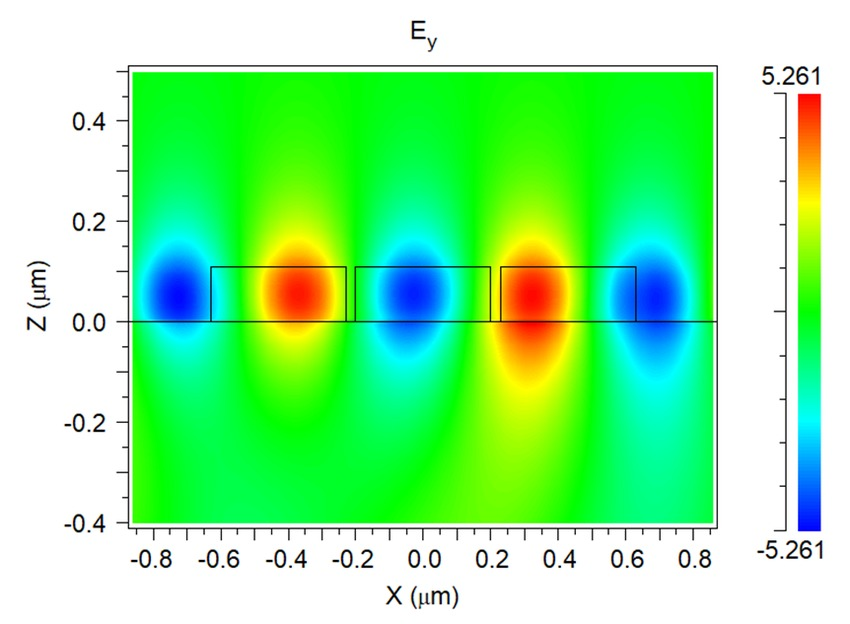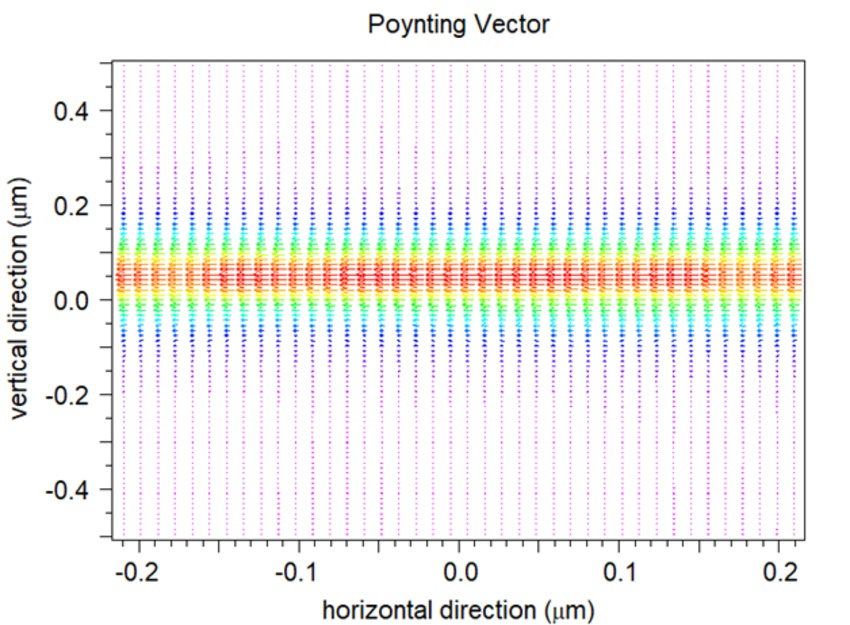Figure 4. The Instantaneous distribution of electric field Ey and Poynting vector at kx = 0.38×2π/ L  (negative GH shift of maximum magnitude).

## References

1. 27 January 2014 | Vol. 22, No. 2 | DOI:10.1364/OE.22.002043 | OPTICS EXPRESS 2043